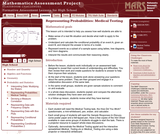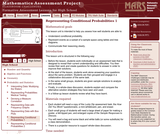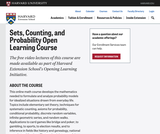# 3 Results

View
Selected filters:
• Conditional ProbabilityOnly Sharing Permitted
CC BY-NC-ND
Rating

This lesson unit is intended to help teachers assess how well students are able to: make sense of a real life situation and decide what math to apply to the problem; understand and calculate the conditional probability of an event A, given an event B, and interpret the answer in terms of a model; represent events as a subset of a sample space using tables, tree diagrams, and Venn diagrams; and interpret the results and communicate their reasoning clearly.

Material Type:
Assessment
Lesson Plan
Provider:
Shell Center for Mathematical Education
U.C. Berkeley
Provider Set:
Mathematics Assessment Project (MAP)
04/26/2013Only Sharing Permitted
CC BY-NC-ND
Rating

This lesson unit is intended to help teachers assess how well students are able to: Understand conditional probability; represent events as a subset of a sample space using tables and tree diagrams; and communicate their reasoning clearly.

Material Type:
Assessment
Lesson Plan
Provider:
Shell Center for Mathematical Education
U.C. Berkeley
Provider Set:
Mathematics Assessment Project (MAP)
04/26/2013Rating

This online math course develops the mathematics needed to formulate and analyze probability models for idealized situations drawn from everyday life. Topics include elementary set theory, techniques for systematic counting, axioms for probability, conditional probability, discrete random variables, infinite geometric series, and random walks. Applications to card games like bridge and poker, to gambling, to sports, to election results, and to inference in fields like history and genealogy, national security, and theology. The emphasis is on careful application of basic principles rather than on memorizing and using formulas.

Material Type:
Homework/Assignment
Lecture